Last updated: 2019-05-27

Checks: 6 0

Knit directory: W_shredder/

This reproducible R Markdown analysis was created with workflowr (version 1.3.0). The Checks tab describes the reproducibility checks that were applied when the results were created. The Past versions tab lists the development history.

Great! Since the R Markdown file has been committed to the Git repository, you know the exact version of the code that produced these results.

Great job! The global environment was empty. Objects defined in the global environment can affect the analysis in your R Markdown file in unknown ways. For reproduciblity it’s best to always run the code in an empty environment.

The command set.seed(20180716) was run prior to running the code in the R Markdown file. Setting a seed ensures that any results that rely on randomness, e.g. subsampling or permutations, are reproducible.

Great job! Recording the operating system, R version, and package versions is critical for reproducibility.

Nice! There were no cached chunks for this analysis, so you can be confident that you successfully produced the results during this run.

Great! You are using Git for version control. Tracking code development and connecting the code version to the results is critical for reproducibility. The version displayed above was the version of the Git repository at the time these results were generated.

Note that you need to be careful to ensure that all relevant files for the analysis have been committed to Git prior to generating the results (you can use wflow_publish or wflow_git_commit). workflowr only checks the R Markdown file, but you know if there are other scripts or data files that it depends on. Below is the status of the Git repository when the results were generated:


Ignored files:
Ignored:    .DS_Store
Ignored:    .Rhistory
Ignored:    .Rproj.user/
Ignored:    Proc_B_manuscript/.DS_Store
Ignored:    analysis/figure/
Ignored:    data/sim_results/
Ignored:    figures/.DS_Store
Ignored:    submission_pack/.DS_Store



Note that any generated files, e.g. HTML, png, CSS, etc., are not included in this status report because it is ok for generated content to have uncommitted changes.

These are the previous versions of the R Markdown and HTML files. If you’ve configured a remote Git repository (see ?wflow_git_remote), click on the hyperlinks in the table below to view them.

File Version Author Date Message
Rmd ea036e3 lukeholman 2019-05-27 All runs finished
Rmd 4f53e5f lukeholman 2019-05-20 pushing parameters_left_to_run change
html 7023078 lukeholman 2019-05-09 Build site.
Rmd e857f2f lukeholman 2019-05-09 wflow_publish(files = "*")
html 80ecff7 lukeholman 2019-05-07 Build site.
Rmd 46a5660 lukeholman 2019-05-07 wflow_publish(files = "*")
html 2278c74 lukeholman 2019-05-07 Build site.
Rmd f7636e1 lukeholman 2019-05-07 wflow_publish(files = "*")
html 0a4b2b8 lukeholman 2019-05-07 Build site.
Rmd 86a1312 lukeholman 2019-05-07 wflow_publish(files = "*")
html f09b255 lukeholman 2019-05-07 Build site.
Rmd 732d19b lukeholman 2019-05-07 wflow_publish(files = "*")
html 38ab91e lukeholman 2019-05-07 Build site.
html bf89903 lukeholman 2019-05-06 Build site.
Rmd 67e9e8e lukeholman 2019-05-06 wflow_publish(files = "*")
html 9c1e80f lukeholman 2019-05-06 Build site.
Rmd 4af9cc2 lukeholman 2019-05-06 wflow_publish(files = "*")
html 780ea2c lukeholman 2019-05-06 Build site.
Rmd 8c3f4f0 lukeholman 2019-05-06 wflow_publish(files = "*")
html 29cf3c2 lukeholman 2019-05-06 Build site.
Rmd 610a420 lukeholman 2019-05-06 wflow_publish(files = "*")
html 2146e95 lukeholman 2019-05-06 Build site.
Rmd c5cedc1 lukeholman 2019-05-06 wflow_publish(files = "*")
html ad7f284 lukeholman 2019-05-06 Build site.
Rmd e16a419 lukeholman 2019-05-06 wflow_publish(files = "*")
html 5a70362 lukeholman 2019-05-06 Build site.
Rmd 881beb7 lukeholman 2019-05-06 wflow_publish(files = "*")
html 881beb7 lukeholman 2019-05-06 wflow_publish(files = "*")
Rmd 80347de lukeholman 2019-04-26 new Proc B version
Rmd 97abdcf lukeholman 2019-04-24 writing and new data finally done
Rmd 76dc4a7 lukeholman 2019-04-15 writing in March
Rmd 5aea3e0 Luke Holman 2019-03-06 tweak future library
Rmd 2160459 Luke Holman 2019-03-06 work in March
html 2160459 Luke Holman 2019-03-06 work in March
Rmd 4fdad66 Luke Holman 2019-02-06 Fixed up plots
html 4fdad66 Luke Holman 2019-02-06 Fixed up plots
Rmd 3b6cc7a Luke Holman 2019-02-05 Making graphs, fixed combine file
Rmd 76f3d92 Luke Holman 2019-01-09 added line to remove cost_A mistake
Rmd 8322107 Luke Holman 2019-01-08 Fix annoying costA+B typo
Rmd 09b03be Luke Holman 2018-12-29 tweaks on desktop
Rmd 15558a7 Luke Holman 2018-12-23 Commit from desktop
Rmd 84db6b2 Luke Holman 2018-12-03 No procedure for checking completed runs
Rmd 2efe487 Luke Holman 2018-11-30 New script to combine files and some writing
Rmd 79a4634 Luke Holman 2018-11-21 Change counting of old parameters
Rmd ee185ed Luke Holman 2018-11-19 Extra params
Rmd de9e0ff Luke Holman 2018-11-13 Added slurm capacity
Rmd 99e93c7 Luke Holman 2018-11-03 Many bug fixes with density dependence
html 99e93c7 Luke Holman 2018-11-03 Many bug fixes with density dependence

packages <- c("dplyr", "purrr", "ggplot2", "reshape2", "Cairo", "knitr", "stringr",
"latex2exp", "pander", "grid", "gridExtra", "ggthemes", "data.table",
"readr", "tibble", "biglm", "kableExtra", "future", "future.apply")
shh <- suppressMessages(lapply(packages, library, character.only = TRUE, quietly = TRUE))

if(!file.exists("data/all_results.rds")){

files <- list.files(path = file.path(getwd(), "data"), pattern = "results_", full.names = TRUE)
files <- files[str_detect(files, "results_[:digit:]+.rds")]
n_files <- length(files)

# Open all the results files and bind them together into a tibble
results <- lapply(1:length(files),
function(i) {
if(i %% 10 == 0) print(paste("Doing file", i, "of", n_files))
readRDS(files[i]) %>% filter(cost_A == 0 & cost_B == 0)}) %>%
rbindlist() %>% as_tibble()

# Make sure each unique parameter space is run exactly once,
# unless it was expressly run multiple times using "realisations" (never used in this paper)
results <- results %>%
distinct(release_strategy, W_shredding_rate, Z_conversion_rate,
Zr_creation_rate, Zr_mutation_rate, Wr_mutation_rate,
cost_Zdrive_female, cost_Zdrive_male, male_migration_prob,
female_migration_prob, migration_type, n_patches, softness,
male_weighting, density_dependence_shape, max_fecundity, realisations,
initial_A, initial_B, .keep_all = TRUE)

saveRDS(results, file = "data/all_results.rds")

results$went_extinct <- ifelse(results$outcome == "Population extinct", 1, 0)
results$migration_type[results$migration_type == "local"] <- "Local"
results$migration_type[results$migration_type == "global"] <- "Global"

# Command to upload the results file to Spartan - needed for Spartan to queue up all the parameter spaces not already finished
# scp /Users/lholman/Rprojects/W_shredder/data/all_results.rds lukeholman@spartan:/data/projects/punim0243/W_shredder/data/all_results.rds

# Helper function to get a list of all the model parameters that vary between runs
find_variable_parameters <- function(dat){
dat %>%
select(-id, -realisations, -generation_extinct, -generation_Zd_extinct,
-generation_W_extinct, -generation_Zd_fixed, -outcome, -went_extinct,
-mating_table, -initial_Wr, -initial_Zr) %>%
sapply(function(x) length(unique(x))) %>%
keep(~.x > 1) %>% names()
}
variable_parameters <- find_variable_parameters(results)
combinations <- apply(combn(variable_parameters, 2), 2, paste0, collapse = " x ") # all 2-way combos of parameters

# Make a data frame to convert R-friendly names to figure-friendly names
nice_names <- data.frame(
original = c(variable_parameters, combinations),
new = gsub("_", " ", c(variable_parameters, combinations)),
stringsAsFactors = FALSE) %>%
mutate(
new = gsub("rel", "Rel", new),
new = gsub("W shredding rate", "Gene drive in females ($p_{shred}$)", new),
new = gsub("Z conversion rate", "Gene drive in males ($p_{conv}$)", new),
new = gsub("Zr creation rate", "Rate of NHEJ ($p_{nhej}$)", new),
new = gsub("Zr mutation rate", "Z-linked resistance ($\\mu{_Z}$)", new),
new = gsub("Wr mutation rate", "W-linked resistance ($\\mu{_W}$)", new),
new = gsub("cost Zdrive female", "Cost of Z* to females ($c_f$)", new),
new = gsub("cost Zdrive male", "Cost of Z* to males ($c_m$)", new),
new = gsub("male migration prob", "Male dispersal frequency", new),
new = gsub("feMale dispersal frequency", "Female dispersal frequency (xf)", new),
new = gsub("Male dispersal frequency", "Male dispersal frequency (xm)", new),
new = gsub("xm", "$x_m$", new),
new = gsub("xf", "$x_f$", new),
new = gsub("migration type", "Dispersal type", new),
new = gsub("n patches", "Number of patches ($k$)", new),
new = gsub("softness", "Importance of local density ($\\psi$)", new),
new = gsub("male weighting", "Male effect on density ($\\delta$)", new),
new = gsub("density dependence shape", "Shape of density dependence ($\\alpha$)", new),
new = gsub("max fecundity", "Maximum fecundity ($r$)", new),
new = gsub("initial A", "Initial freq. W-shredding resistance allele A", new),
new = gsub("initial B", "Initial freq. gene conversion resistance allele B", new),
new = as.character(TeX(new))) %>%
mutate(new = gsub("mu", "\\mu", new))

# Sort the results between those that model a W-shredder, versus those that model a Z* that sterilises females
W_shredder <- results %>% filter(cost_Zdrive_female != 1)
female_sterilising <- results %>% filter(cost_Zdrive_female == 1)

pretty <- function(x) prettyNum(x, big.mark = ",", scientific = FALSE)

# Count the number of runs and save for entry into the manuscript
data.frame(total_runs = pretty(nrow(results)),
W_shredder_runs = pretty(nrow(W_shredder)),
female_sterilising_runs = pretty(nrow(female_sterilising)),
W_shredder_runs_extinct = pretty(sum(W_shredder$went_extinct)), female_sterilising_runs_extinct = pretty(sum(female_sterilising$went_extinct)))  %>%
saveRDS(file = "data/number_of_runs.rds")

rm(results)

## Table showing the frequencies of each possible outcome

Table S1: The number and percentage of simulation runs that ended with the five possible outcomes, for the subset of simulation runs focusing on a W-shredder gene drive.

make_tally_table <- function(dat){
outcomes <- dat$outcome %>% table() %>% melt() %>% arrange(-value) %>% mutate(p = round(100 * value / sum(value), 1)) names(outcomes) <- c("Outcome", "Number of simulations", "%") outcomes %>% mutate(Outcome = gsub("without", "without causing", Outcome), Outcome = gsub("n extinct", "n went extinct", Outcome), Outcome = gsub("d extinct", "d went extinct", Outcome), Outcome = gsub("Zd", "Z*", Outcome)) } table_S1 <- make_tally_table(W_shredder) saveRDS(table_S1, file = "figures/tableS1.rds") table_S1 %>% pander() Outcome Number of simulations % Z* fixed without causing extinction 2466751 41.3 Z* went extinct 1686105 28.2 Population went extinct 950839 15.9 Wr fixed 725615 12.2 Timer expired 142658 2.4 Table S2: The number and percentage of simulation runs that ended with the five possible outcomes, for the subset of simulation runs focusing on a female-sterilising Z-linked gene drive. table_S2 <- make_tally_table(female_sterilising) saveRDS(table_S2, file = "figures/tableS2.rds") table_S2 %>% pander() Outcome Number of simulations % Z* went extinct 569698 85.9 Timer expired 73224 11 Population went extinct 13371 2 Wr fixed 7259 1.1 ## Make Figure 2 plot_run <- function(){ get_data <- function(model_id, allele_freqs_list, result_df, label){ df <- pluck_allele_freqs(model_id, allele_freqs_list) alleles_to_plot <- group_by(df, allele) %>% summarise(uniques = length(unique(frequency))) %>% filter(uniques > 1) %>% # Don't plot alleles that stay at 0 whole time pull(allele) alleles_to_plot <- c(alleles_to_plot, "N") df <- df[df$allele %in% alleles_to_plot, ]
df$frequency[df$allele == "N"] <- df$frequency[df$allele == "N"] /
max(df$frequency[df$allele == "N"])

paras <- result_df %>% mutate(id = as.character(id)) %>% filter(id == model_id)
last_generation <- tail(df,12) %>% select(allele, frequency)
print(paras); print(last_generation)

df %>% mutate(facet = label,
allele = replace(allele, allele == "Zd", "Z*"),
allele = replace(allele, allele == "females", "Females"))
}

rbind(
get_data("10024119427447", allele_freqs, W_shredder, "A. Z* causes rapid extinction"),
get_data("1002596940286", allele_freqs, W_shredder, "B. Z* fails to cause extinction"),
get_data("10024112958792", allele_freqs, W_shredder, "C. Resistance to Z* prevents extinction")) %>%
ggplot(aes(x = generation, y = frequency, colour = allele, group = allele)) +
geom_vline(xintercept = 50, linetype = 3, colour = "grey10", size = 0.9) +
geom_line(size = 0.9, alpha = 0.9) +
facet_wrap(~facet, scales = "free_x") +
theme_hc() + scale_colour_hc(name = "") +
theme(strip.text = element_text(hjust = 0),
strip.background = element_rect(fill = "grey90"),
legend.position = "top",
axis.ticks.y = element_blank()) +
xlab("Generation") + ylab("Frequency")
}

fig2 <- plot_run()
fig2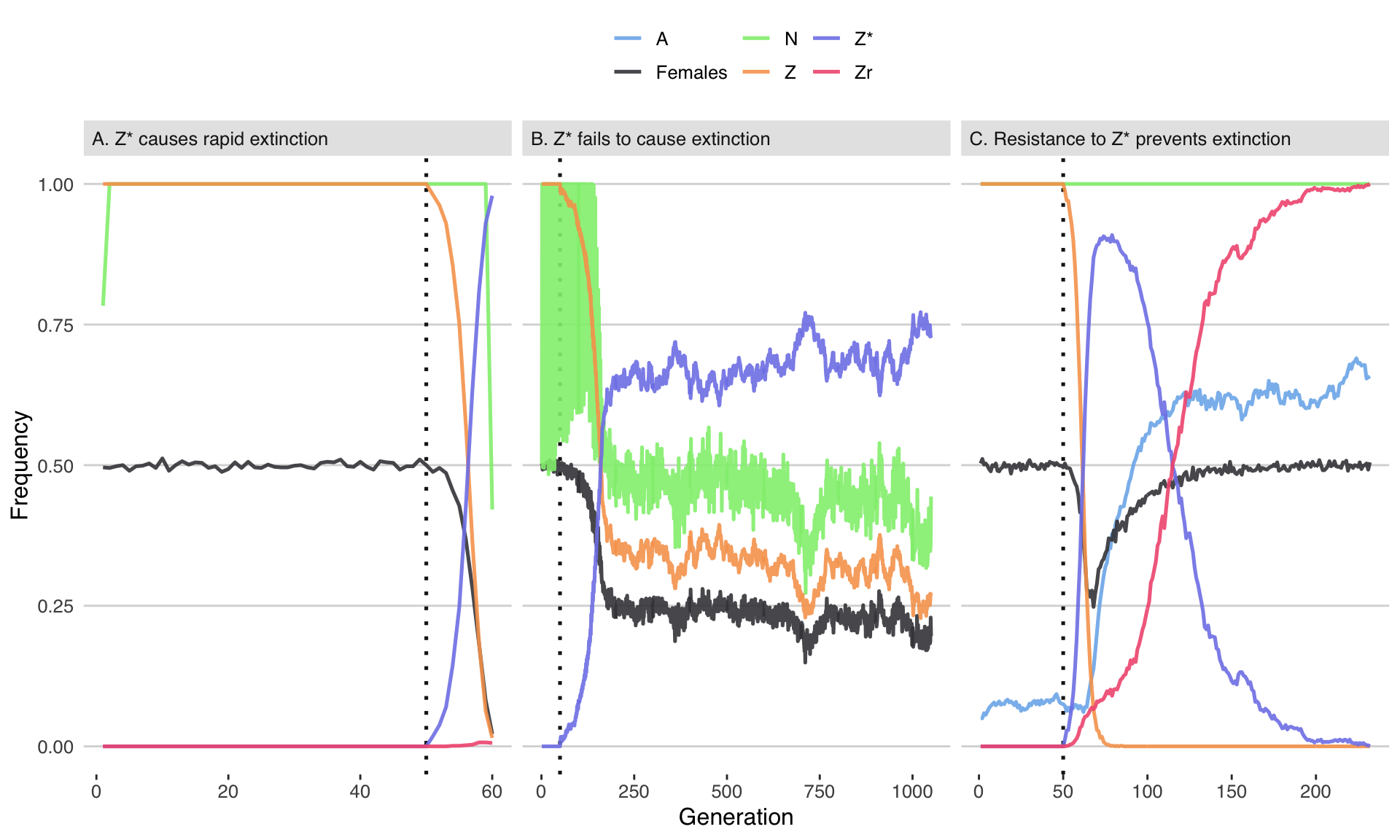Version Author Date
29cf3c2 lukeholman 2019-05-06

Figure 2: Three illustrative runs of the simulation, showing evolution in response to the introduction of 20 males carrying a W-shredder at Generation 50 (marked by the dotted line). In panel A, the driving Z* allele fixed very quickly, causing population extinction as the number of females dropped to zero. In panel B, the Z* allele spread up until the point that its fitness costs began to negate its transmission advantage, causing the population to halve in size but not to go extinct. In panel C, the Z* allele invaded, selecting for the resistance alleles A and Zr, and causing the Z* allele to reverse course and go extinct. The population size N is shown as a fraction of its maximum value of 10,000. Table S3 gives the parameter spaces used for these three runs.

table_S3 <- t(rbind(
data.frame(Panel = "A", W_shredder %>% filter(id == "10024119427447")),
data.frame(Panel = "B", W_shredder %>% filter(id == "1002596940286")),
data.frame(Panel = "C", W_shredder %>% filter(id == "10024112958792"))) %>%
select(release_strategy, W_shredding_rate,
Z_conversion_rate, Zr_creation_rate, Zr_mutation_rate,
Wr_mutation_rate, cost_Zdrive_female, cost_Zdrive_male,
male_migration_prob, female_migration_prob,
migration_type, n_patches, softness, male_weighting,
density_dependence_shape, max_fecundity, initial_A, initial_B))

nice_names2 <- data.frame(original = variable_parameters,
new = gsub("_", " ", variable_parameters),
stringsAsFactors = FALSE) %>%
mutate(
new = gsub("rel", "Rel", new),
new = gsub("W shredding rate", "Gene drive in females (p_shred)", new),
new = gsub("Z conversion rate", "Gene drive in males (p_conv)", new),
new = gsub("Zr creation rate", "Rate of NHEJ (p_nhej)", new),
new = gsub("Zr mutation rate", "Z-linked resistance (μ_Z)", new),
new = gsub("Wr mutation rate", "W-linked resistance (μ_W)", new),
new = gsub("cost Zdrive female", "Cost of Z* to females (c_f)", new),
new = gsub("cost Zdrive male", "Cost of Z* to males (c_m)", new),
new = gsub("male migration prob", "Male dispersal frequency", new),
new = gsub("feMale dispersal frequency", "Female dispersal frequency (x_f)", new),
new = gsub("Male dispersal frequency", "Male dispersal frequency (x_m)", new),
new = gsub("migration type", "Dispersal type", new),
new = gsub("n patches", "Number of patches (k)", new),
new = gsub("softness", "Importance of local density (ψ)", new),
new = gsub("male weighting", "Male effect on density (𝛿)", new),
new = gsub("density dependence shape", "Shape of density dependence (α)", new),
new = gsub("max fecundity", "Maximum fecundity (r)", new),
new = gsub("initial A", "Intial freq. W-shredding resistance allele A", new),
new = gsub("initial B", "Intial freq. gene conversion resistance allele B", new))

rownames(table_S3) <- nice_names2$new[match(rownames(table_S3), nice_names2$original)]
rownames(table_S3) <- gsub("[.]", "", rownames(table_S3))
colnames(table_S3) <- c("Panel A", "Panel B", "Panel C")
table_S3[table_S3 == "one_patch"] <- "All in one patch"
table_S3[table_S3 == "all_patches"] <- "Scattered over all patches"

Table S3: List of the parameter values used to generate the simulation runs shown in Figure 2.

as.data.frame(table_S3) %>% kable(format = "html", escape = FALSE) %>% kable_styling()
Panel A Panel B Panel C
Release strategy All in one patch All in one patch Scattered over all patches
Gene drive in females (p_shred) 1 1 1
Gene drive in males (p_conv) 0.95 0.00 0.50
Rate of NHEJ (p_nhej) 0.01 0.00 0.10
Z-linked resistance (μ_Z) 0 0 0
W-linked resistance (μ_W) 0 0 0
Cost of Z* to females (c_f) 0.01 0.50 0.50
Cost of Z* to males (c_m) 0.01 0.20 0.01
Male dispersal frequency (x_m) 0.50 0.05 0.05
Female dispersal frequency (x_f) 0.5 0.5 0.5
Dispersal type Global Global Global
Number of patches (k) 20 20 20
Importance of local density (ψ) 1 1 1
Male effect on density (𝛿) 1.0 1.5 1.0
Shape of density dependence (α) 1.0 0.2 1.8
Maximum fecundity (r) 50 50 50
Intial freq W-shredding resistance allele A 0.00 0.00 0.05
Intial freq gene conversion resistance allele B 0 0 0

## Plot the effects of each parameter on extinction

# Find the proportion of runs that went extinct for each parameter value
get_percent_extinct <- function(dat, parameter){
dat %>%
group_by(!! sym(parameter)) %>%
summarise(extinct = sum(went_extinct),
not_extinct = n() - sum(went_extinct),
prob = list(binom.test(extinct, extinct + not_extinct))) %>%
rowwise() %>%
mutate(response_variable = 100 * extinct/(extinct + not_extinct),
lower_95_CI = 100 * prob$conf.int, upper_95_CI = 100 * prob$conf.int,
parameter =  parameter) %>% rename(value = !! sym(parameter)) %>%
select(parameter, value, everything()) %>% select(-prob) %>% ungroup()
}

# Find the median time to extinction, among just those runs in which extinction occurred, for each parameter value
get_extinction_time <- function(dat, parameter){
dat %>%
filter(!is.na(generation_extinct)) %>% # only include runs where extinction occurred
group_by(!! sym(parameter)) %>%
summarise(response_variable = median(generation_extinct),
lower_95_CI = quantile(generation_extinct, probs = 0.025),
upper_95_CI = quantile(generation_extinct, probs = 0.975),
n = n(),
parameter =  parameter) %>% rename(value = !! sym(parameter)) %>%
select(parameter, value, everything()) %>% ungroup()
}

# To calculate separately for the 3 levels of Z_conversion_rate (male gene drive)
split_by_male_drive <- function(dat, fun){
rbind(
lapply(
variable_parameters[variable_parameters != "Z_conversion_rate"],
get(fun),
dat = dat %>%
filter(Z_conversion_rate == 0)) %>% do.call("rbind", .) %>% mutate(Z_conversion_rate = 0),
lapply(
variable_parameters[variable_parameters != "Z_conversion_rate"],
get(fun),
dat = dat %>%
filter(Z_conversion_rate == 0.5)) %>% do.call("rbind", .) %>% mutate(Z_conversion_rate = 0.5),
lapply(
variable_parameters[variable_parameters != "Z_conversion_rate"],
get(fun),
dat = dat %>%
filter(Z_conversion_rate == 0.95)) %>% do.call("rbind", .) %>% mutate(Z_conversion_rate = 0.95)
) %>% mutate(Z_conversion_rate = factor(Z_conversion_rate, levels = c(0, 0.5, 0.95)))
}

# % extinct, calculated separately for the three levels of Z_conversion_rate
W_shredder_split_by_male_drive_percent_extinct <- split_by_male_drive(W_shredder, "get_percent_extinct")
female_sterilising_split_by_male_drive_percent_extinct <- split_by_male_drive(female_sterilising, "get_percent_extinct") %>%
filter(!(parameter %in% c("cost_Zdrive_female", "W_shredding_rate")))

# Time until extinction, calculated separately for the three levels of Z_conversion_rate
W_shredder_split_by_male_drive_time_to_extinct <- split_by_male_drive(W_shredder, "get_extinction_time")
female_sterilising_split_by_male_drive_time_to_extinct <- split_by_male_drive(female_sterilising, "get_extinction_time") %>%
filter(!(parameter %in% c("cost_Zdrive_female", "W_shredding_rate")))

# Function to make Figure 3 (and similar supplementary figures)
make_multipanel_figure <- function(dat, ylab){
parameter_importance <- dat %>%
mutate(parameter = nice_names$new[match(parameter, nice_names$original)]) %>%
group_by(parameter) %>% summarise(range = max(response_variable) - min(response_variable)) %>%
arrange(-range) %>% pull(parameter)

levels <- levels(factor(dat$value)) levels <- c(levels[!(levels %in% c("all_patches", 100))], 100, "all_patches") levels <- replace(levels, levels == "all_patches", "Release in\nall patches") levels <- replace(levels, levels == "one_patch", "Release in\na single patch") pd <- position_dodge(0.2) dat %>% mutate(parameter = nice_names$new[match(parameter, nice_names$original)], parameter = factor(parameter, parameter_importance), value = replace(value, value == "all_patches", "Release in\nall patches"), value = replace(value, value == "one_patch", "Release in\na single patch"), value = factor(value, levels)) %>% ggplot(aes(value, response_variable, colour = Z_conversion_rate)) + geom_errorbar(aes(ymin = lower_95_CI, ymax = upper_95_CI), width = 0, position = pd) + geom_point(position = pd) + scale_colour_brewer(palette = "Set2", name = "Gene drive in males\n(p_conv)") + xlab("Parameter value") + ylab(ylab) + theme_bw() + theme(strip.background = element_blank(), panel.grid.major.x = element_blank(), panel.border = element_rect(fill = NA, size = 1)) } fig3 <- make_multipanel_figure(W_shredder_split_by_male_drive_percent_extinct, ylab = "% simulation runs resulting in extinction (\u00B1 95% CIs)") ### % extinction plot for a W-shredder (Figure 3) cairo_pdf(file = "figures/figure3.pdf", width = 9, height = 12) fig3 + facet_wrap(~parameter, scales = "free", labeller = label_parsed, ncol = 4) + theme(legend.position = c(.9, .1)) dev.off() quartz_off_screen 2  fig3 + facet_wrap(~parameter, scales = "free", labeller = label_parsed, ncol = 3)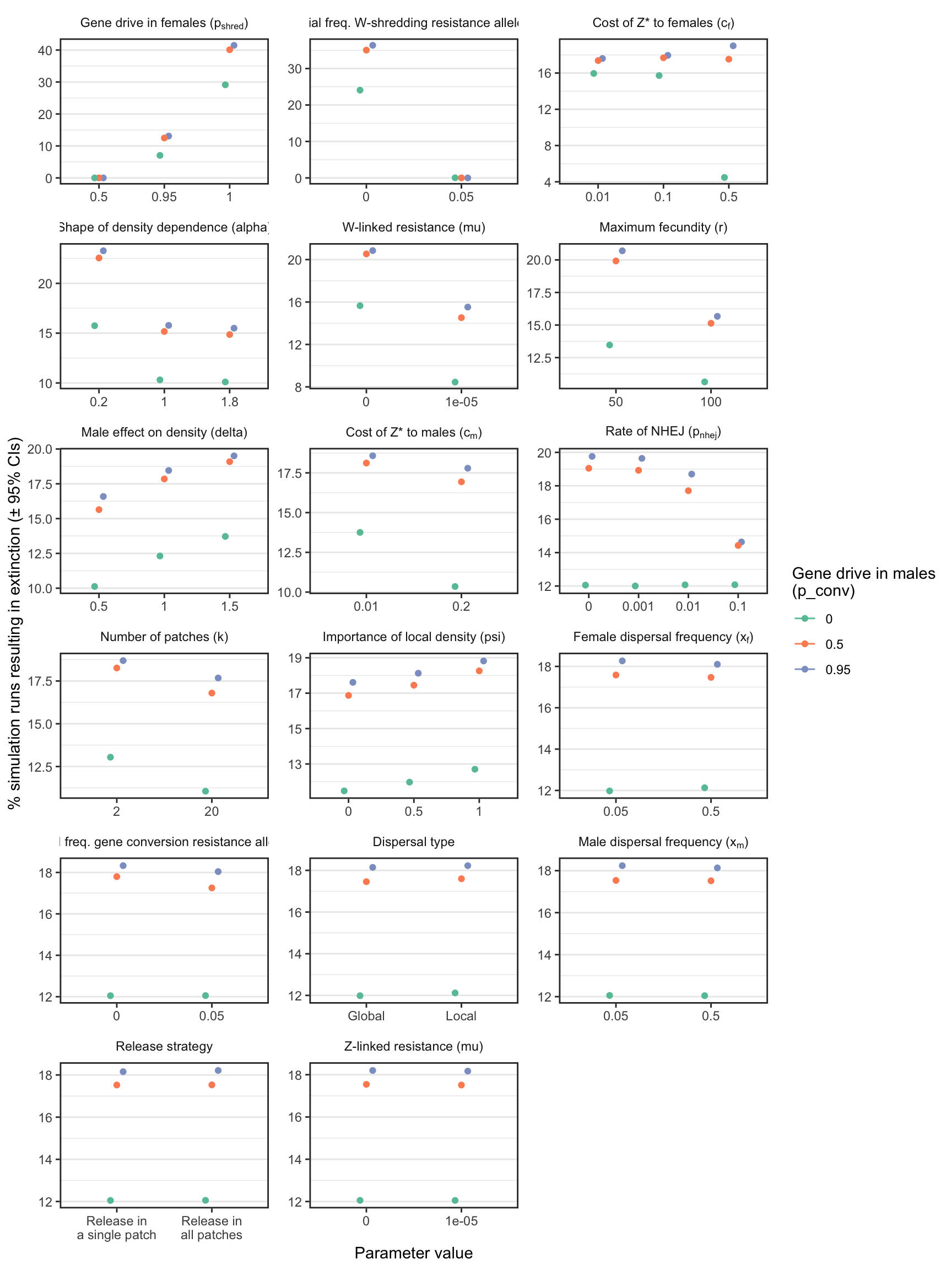Version Author Date 80ecff7 lukeholman 2019-05-07 780ea2c lukeholman 2019-05-06 29cf3c2 lukeholman 2019-05-06 ad7f284 lukeholman 2019-05-06 Figure 3: The percentage of simulations of a W-shredder that ended in extinction, for all runs with a particular value (shown on the $$x$$-axis) for a given parameter (shown in the panels). For example, in all the thousands of runs for which I assumed $$p_{shred} = 0.5$$, there were no extinctions, while among the runs where $$p_{shred} = 1$$, over 60% resulted in extinction. The panels are ordered by the range of the y-axis, which highlights the relative importance of the variables for the probability of extinction. Figure S1 shows the median time-to-extinction for each parameter value, and Figures S3-S4 give similar plots for simulations that considered a female-sterilising Z-linked gene drive instead of a W-shredder. ### Time-to-extinction plot for a W-shredder (Figure S1) S1_fig <- make_multipanel_figure(filter(W_shredder_split_by_male_drive_time_to_extinct, n >= 40), ylab = "Median generations until extinction (\u00B1 95% quantiles)") + facet_wrap(~parameter, scales = "free", labeller = label_parsed, ncol = 3) + coord_cartesian(ylim = c(0, 150)) ggsave(S1_fig, filename = "figures/S1_fig.pdf", height = 12, width = 19.5) saveRDS(S1_fig, file = "figures/S1_fig.rds") S1_fig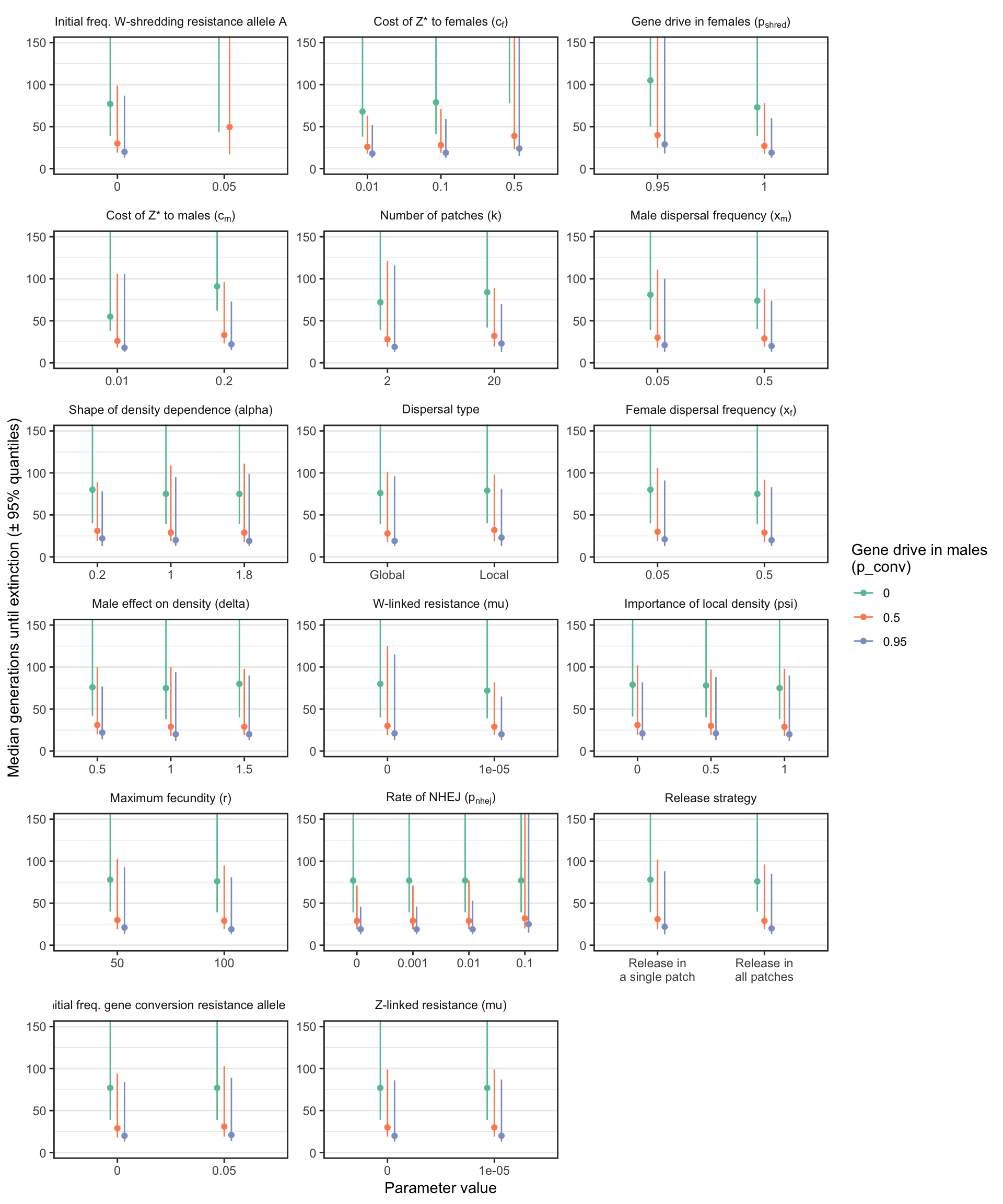Version Author Date 80ecff7 lukeholman 2019-05-07 780ea2c lukeholman 2019-05-06 29cf3c2 lukeholman 2019-05-06 ad7f284 lukeholman 2019-05-06 Figure S1: Similar plot to Figure 3, except that the y-axis shows the median number of generations until the W-shredder caused extinction, among just the subset of simulations in which extinction actually occurred. A median was only calculated if at least 40 simulation runs reached extinction. ### % extinction plot for a female-sterilising Z-drive (Figure S3) S3_fig <- make_multipanel_figure(female_sterilising_split_by_male_drive_percent_extinct, ylab = "% simulation runs resulting in extinction (\u00B1 95% CIs)") + facet_wrap(~parameter, scales = "free", labeller = label_parsed, ncol = 4) ggsave(S3_fig, filename = "figures/S3_fig.pdf", height = 9.6, width = 10) saveRDS(S3_fig, file = "figures/S3_fig.rds") S3_fig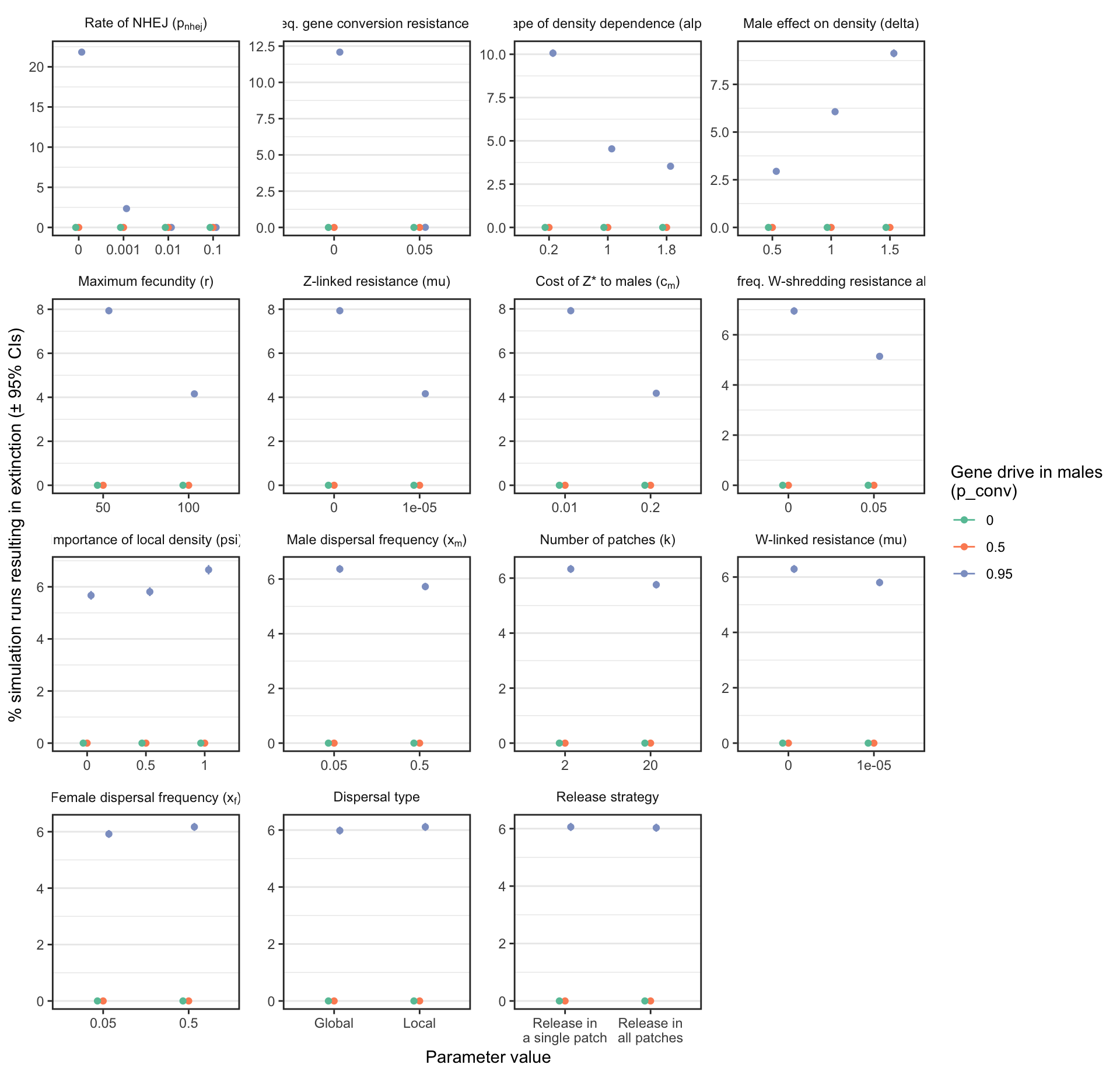Version Author Date 80ecff7 lukeholman 2019-05-07 780ea2c lukeholman 2019-05-06 29cf3c2 lukeholman 2019-05-06 ad7f284 lukeholman 2019-05-06 Figure S3: Analagous information to Figure 3, but showing the results for a female-sterilising Z* allele instead of a W-shredder. ### Time-to-extinction plot for a female-sterilising Z-drive (Figure S4) S4_fig <- make_multipanel_figure(filter(female_sterilising_split_by_male_drive_time_to_extinct, n >= 40), ylab = "Median generations until extinction (\u00B1 95% quantiles)") + facet_wrap(~parameter, scales = "free", labeller = label_parsed, ncol = 3) + coord_cartesian(ylim = c(0, 100)) ggsave(S4_fig, filename = "figures/S4_fig.pdf", height = 12, width = 10) saveRDS(S4_fig, file = "figures/S4_fig.rds") S4_fig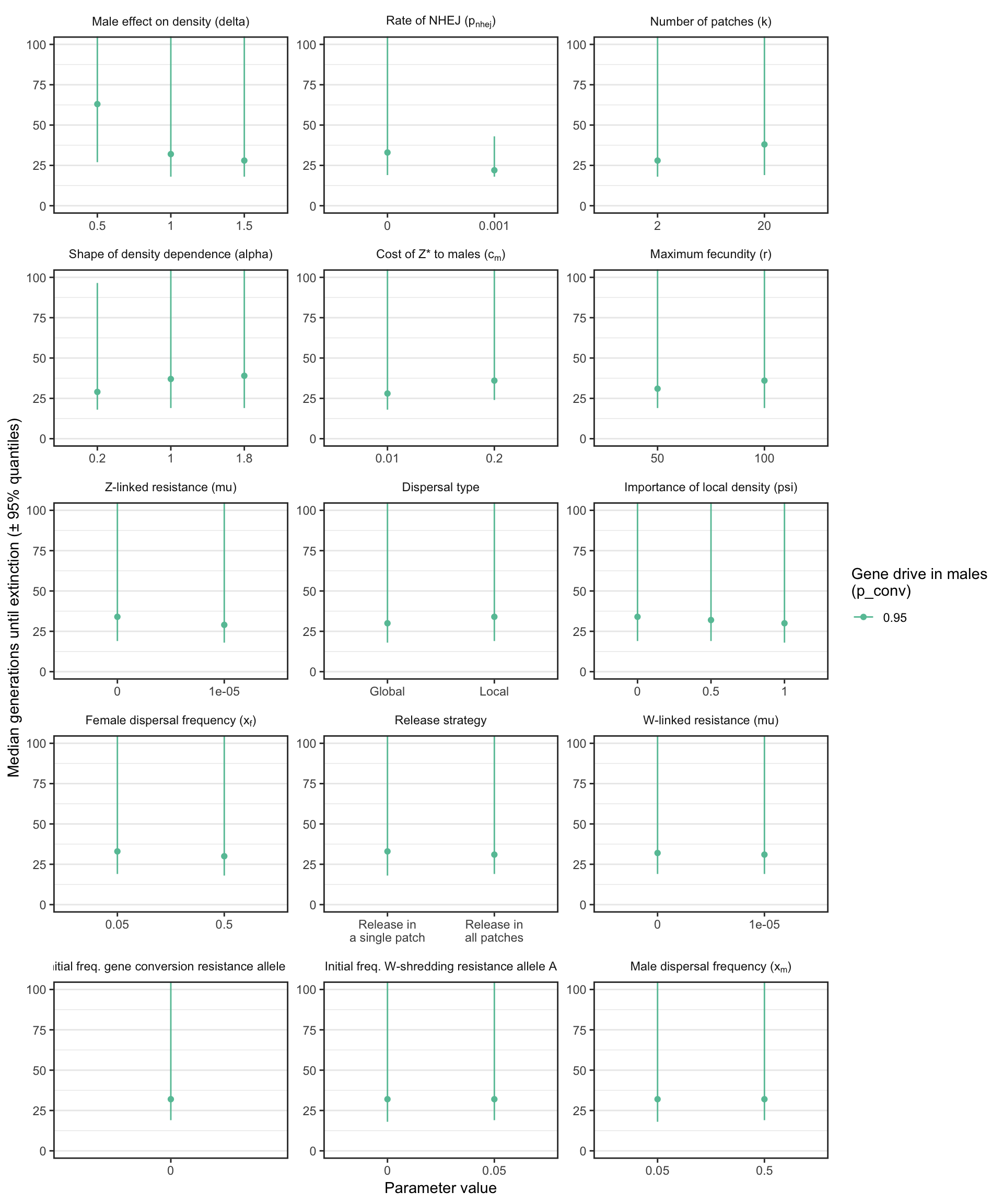Version Author Date 29cf3c2 lukeholman 2019-05-06 ad7f284 lukeholman 2019-05-06 Figure S4: Analagous information to Figure S1, but showing the results for a female-sterilising Z* allele instead of a W-shredder. Note that a median was only calculated if at least 40 simulation runs reached extinction, and extinction only occurred when gene drive in males was strong. ## Finding variables with interacting effects on the extinction probability ### Testing for interactions using GLM Run a binomial generalized linear model (GLM), with all of the variable model parameters and all their 2-way interactions as predictors. The response variable is extinction, coded as a zero or one. Since the number of data points is very large (millions), I use the biglm package (“big GLM”). I run a model separately on all the runs that considered the evolution of a W-shredder, and all the runs that considered a female-sterilising Z*. run_big_glm <- function(dat, sterilising = FALSE){ if(sterilising){ variable_parameters <- variable_parameters[!(variable_parameters %in% c("W_shredding_rate", "cost_Zdrive_female"))] } formula <- paste("went_extinct ~ (", paste0(variable_parameters, collapse = " + "), ")^2", sep = "") if(!sterilising) formula <- paste(formula, "- W_shredding_rate:cost_Zdrive_female") bigglm(as.formula(formula), data = dat %>% mutate_if(is.numeric, function(x) as.numeric(scale(x))), chunksize = 10000) } # Helper to get the fixed effects table out of the biglm object (no 'coefficients' slot, apparently) tidy_model <- function(model){ out <- capture.output(model %>% summary)[-(1:5)] %>% lapply(function(x) { x <- strsplit(x, split = " ")[] x[x != ""] }) %>% do.call("rbind", .) %>% as.data.frame(stringsAsFactors = FALSE) %>% as_tibble() %>% mutate(V1 = as.factor(gsub(":", " x ", V1))) %>% mutate_if(is.character, as.numeric) names(out) <- c("Parameter", "Estimate", "Lower_95_CI", "Upper_95_CI", "SE", "p") out %>% arrange(-abs(Estimate)) } if(!file.exists("data/W_shredder_model.rds")) { W_shredder_model <- run_big_glm(W_shredder) saveRDS(tidy_model(W_shredder_model), "data/W_shredder_model.rds") female_sterilising_model <- run_big_glm(female_sterilising, sterilising = TRUE) saveRDS(tidy_model(female_sterilising_model), "data/female_sterilising_model.rds") } else { W_shredder_model <- readRDS("data/W_shredder_model.rds") female_sterilising_model <- readRDS("data/female_sterilising_model.rds") } ### Finding the top-ranked effects in the GLM importance_plot <- function(dat, n = "all"){ if(n != "all"){ dat <- dat %>% mutate(row=1:n()) %>% filter(row %in% 1:n) } for(i in 1:nrow(dat)){ if(dat$Estimate[i] < 0){
lower <- dat$Lower_95_CI[i] dat$Lower_95_CI[i] <- dat$Upper_95_CI[i] dat$Upper_95_CI[i] <- lower
}
}

dat <- dat %>%
mutate(Parameter = gsub("one_patch", "", as.character(Parameter)),
Parameter = gsub("Local", "", Parameter),
Parameter = factor(Parameter, rev(Parameter)),
tick_label = nice_names$new[match(Parameter, nice_names$original)])

dat %>%
ggplot(aes(Parameter, abs(Estimate))) +
geom_bar(stat = "identity", fill = "tomato") +
geom_errorbar(aes(ymin = abs(Lower_95_CI), ymax = abs(Upper_95_CI)), width = 0) +
coord_flip() +
scale_y_continuous(expand = c(0, 0)) +
scale_x_discrete(labels = parse(text = rev(dat$tick_label))) + xlab("Predictor variable") + ylab("Absolute effect size (\u00B1 95% CIs)") + theme_classic() + theme(axis.ticks.y = element_blank()) } W_shred <- importance_plot(W_shredder_model, n = 25) female_sterile <- importance_plot(female_sterilising_model, n = 25) #### For a W-shredder (Figure 4) W_shred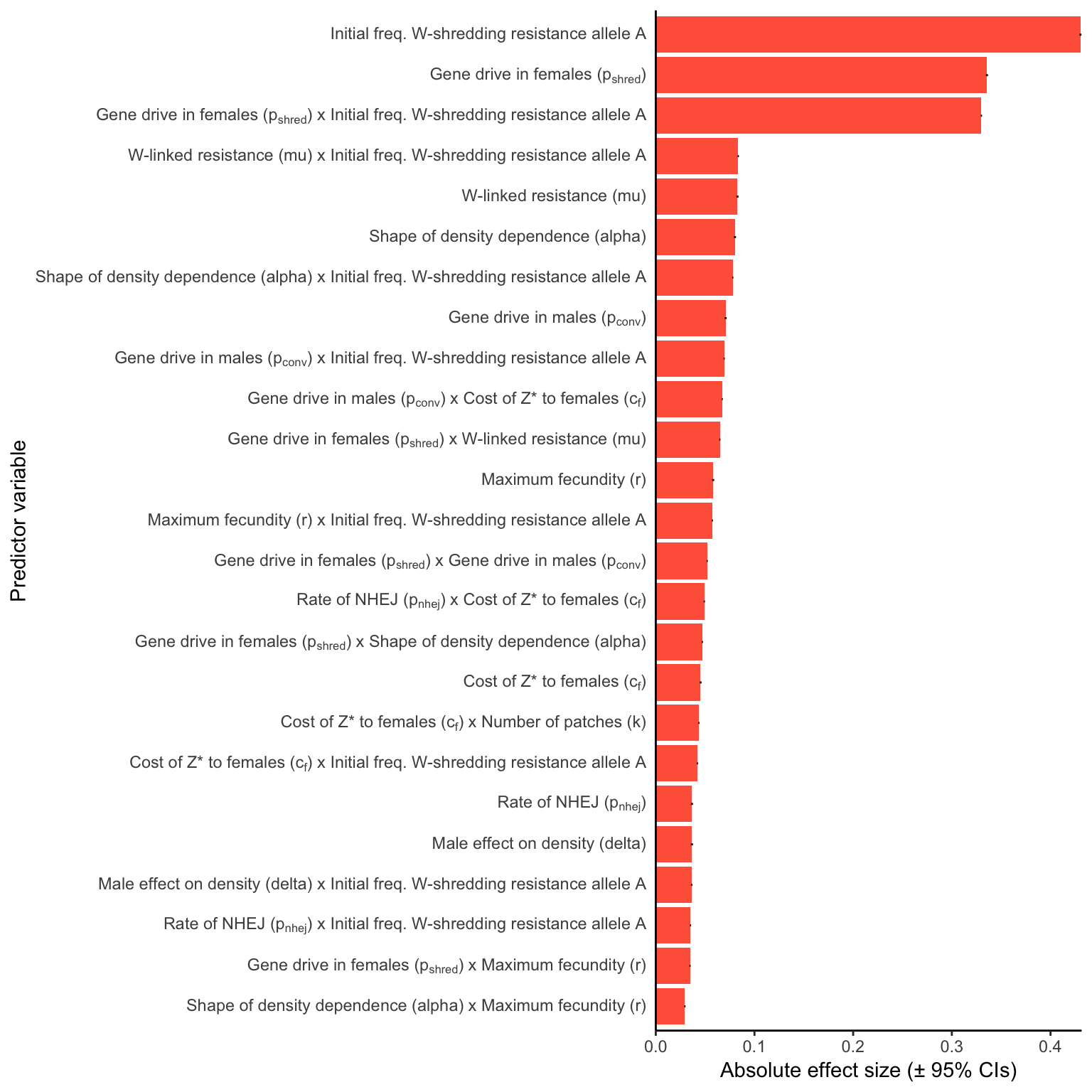Figure 4: Relative parameter importance in the simulations of W-shredders, for the top 25 most important main effects or two-way interactions (from a binomial GLM that included all the main effects and all their two-way interactions). Each predictor variable was scaled before running the model, meaning that the absolute effect size indicates how important each parameter is to the extinction probability, given the range of values plotted in Figure 3. Figure S5 gives a similar plot for simulations of a female-sterilising $$Z^*$$ allele. #### For a female-sterilising drive female_sterile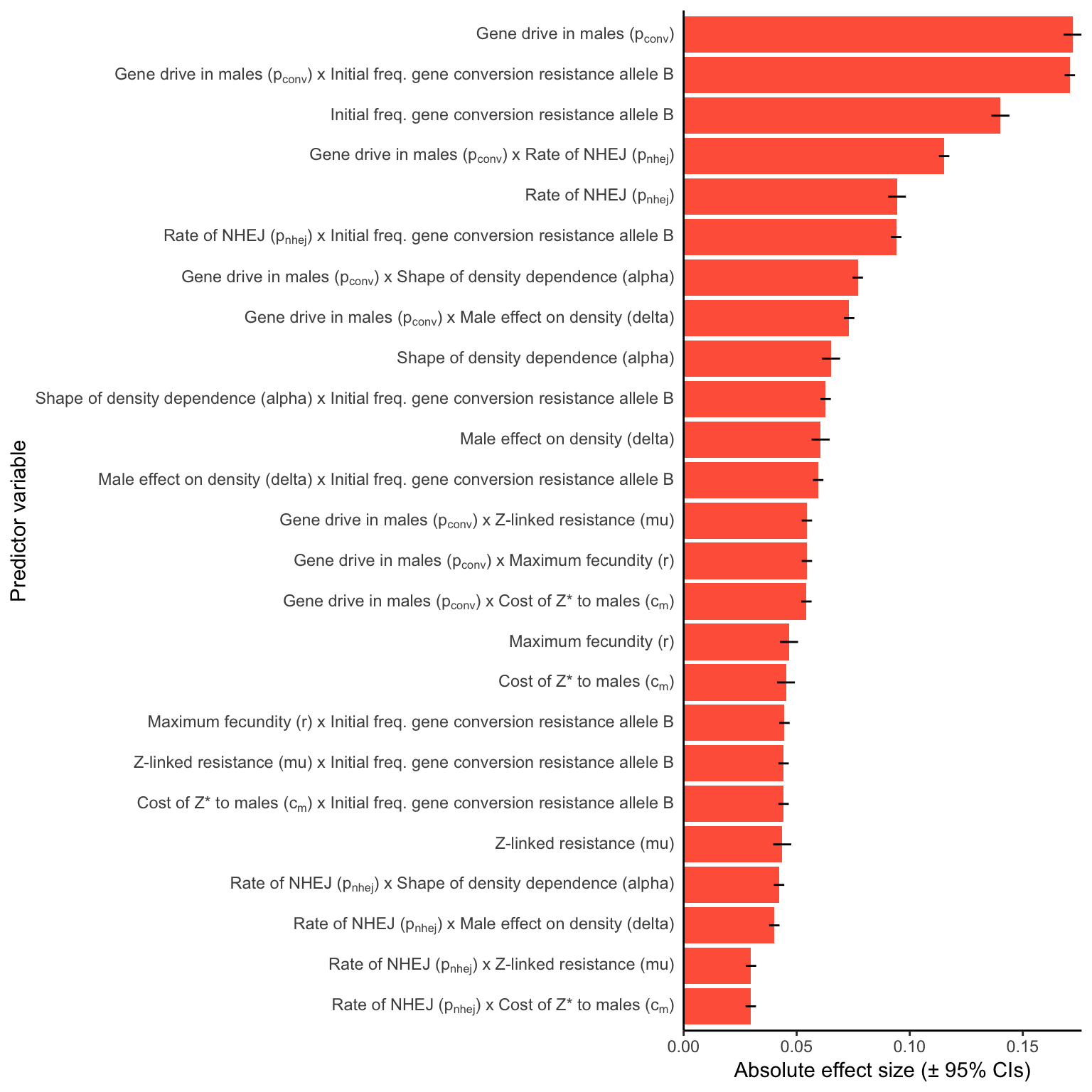Figure S5: Relative parameter importance in the simulations of Z-linked female-sterilising gene drives, for the top 25 most important main effects or two-way interactions (from a binomial GLM that included all the main effects and all their two-way interactions). Each predictor variable was scaled before running the model, meaning that the absolute effect size indicates how important each parameter is to the extinction probability, given the range of values plotted in Figure S3. ### Plot showing interacting effects on extinction probability get_percent_extinct_double <- function(dat, p1, p2){ output <- dat %>% group_by(!! sym(p1), !! sym(p2)) %>% summarise(extinct = sum(went_extinct), not_extinct = n() - sum(went_extinct), prob = list(binom.test(extinct, extinct + not_extinct))) %>% rowwise() %>% mutate(percent_extinct = 100 * extinct / (extinct + not_extinct), lower_95_CI = 100 * prob$conf.int,
upper_95_CI = 100 * prob$conf.int, parameter_1 = p1, parameter_2 = p2) names(output)[1:2] <- paste("value", 1:2, sep = "_") output %>% mutate(value_1 = as.character(value_1), value_2 = as.character(value_2)) %>% select(parameter_1, parameter_2, value_1, value_2, everything()) %>% select(-prob) %>% ungroup() } top_12_shredder <- (W_shredder_model %>% mutate(Parameter = as.character(Parameter)) %>% filter(grepl(" x ", Parameter)) %>% pull(Parameter))[1:12] top_12_sterilising <- (female_sterilising_model %>% mutate(Parameter = as.character(Parameter)) %>% filter(grepl(" x ", Parameter)) %>% pull(Parameter))[1:12] two_way_W_shredder <- map2_df(str_split(top_12_shredder, " x ", simplify = TRUE)[, 1], str_split(top_12_shredder, " x ", simplify = TRUE)[, 2], get_percent_extinct_double, dat = W_shredder) two_way_sterilising <- map2_df(str_split(top_12_sterilising, " x ", simplify = TRUE)[, 1], str_split(top_12_sterilising, " x ", simplify = TRUE)[, 2], get_percent_extinct_double, dat = W_shredder) two_way_plot <- function(two_way_data, pairwise_combos){ two_way_data <- two_way_data %>% mutate(pasted = paste(parameter_1, parameter_2, sep = " x "), parameter_1 = nice_names$new[match(parameter_1, nice_names$original)], parameter_2 = nice_names$new[match(parameter_2, nice_names$original)]) %>% filter(pasted %in% pairwise_combos) z_range <- range(two_way_data$percent_extinct) * 1.1

make_one <- function(df){

df$value_1 <- factor(df$value_1, unique(df$value_1)) df$value_2 <- factor(df$value_2, unique(df$value_2))

ggplot(df, aes(value_1, value_2, fill = percent_extinct)) +
geom_tile(colour = "black", linetype = 3) +
scale_fill_distiller(palette = "PuBuGn", direction = 1, limits = z_range, name = "% extinct") +
theme_bw() +
theme() +
scale_x_discrete(expand = c(0,0), name = parse(text = df$parameter_1)) + scale_y_discrete(expand = c(0,0), name = parse(text = df$parameter_2))

}

grid_arrange_shared_legend <- function(...) {
plots <- list(...)
g <- ggplotGrob(plots[] + theme(legend.position="bottom"))$grobs legend <- g[[which(sapply(g, function(x) x$name) == "guide-box")]]
lheight <- sum(legend\$height)
plots <- lapply(plots, function(x) ggplotGrob(x + theme(legend.position = "none")))

p <- gtable_cbind(gtable_rbind(plots[], plots[], plots[]),
gtable_rbind(plots[], plots[], plots[]),
gtable_rbind(plots[], plots[], plots[]))

grid.arrange(p, legend,
nrow = 2,
heights = unit.c(unit(1, "npc") - lheight, lheight))
}

dat_list <- vector(mode = "list", length = length(pairwise_combos))
for(i in 1:length(pairwise_combos)){
dat_list[[i]] <- two_way_data %>% filter(pasted == pairwise_combos[i])
}

lapply(dat_list, make_one) %>% do.call("grid_arrange_shared_legend", .)
}

two_way_plot_shredder <- two_way_plot(two_way_W_shredder, top_12_shredder)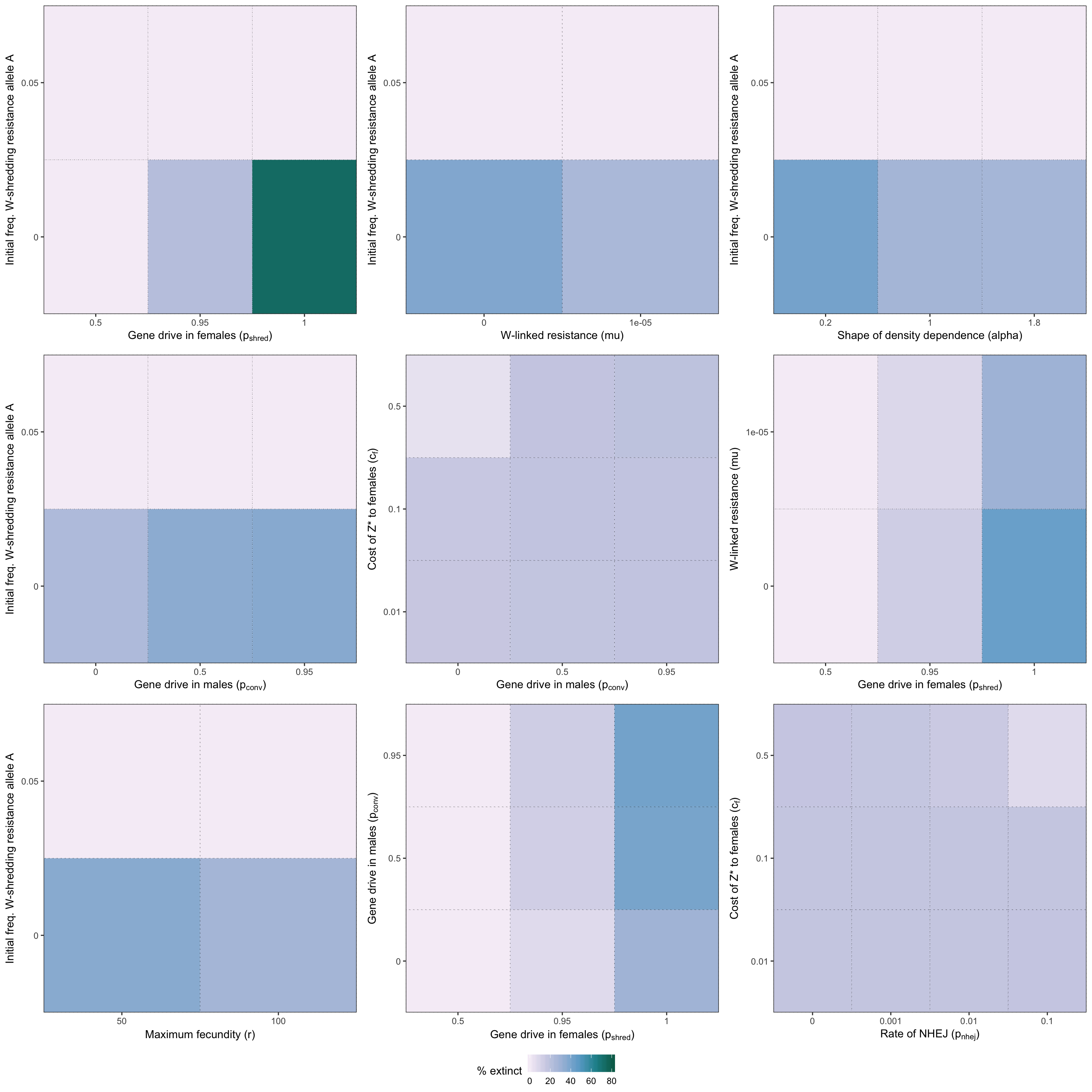Version Author Date
80ecff7 lukeholman 2019-05-07
9c1e80f lukeholman 2019-05-06
two_way_plot_sterilising <- two_way_plot(two_way_sterilising, top_12_sterilising)
saveRDS(two_way_plot_shredder, file = "figures/S2_fig.rds")
saveRDS(two_way_plot_sterilising, file = "figures/S6_fig.rds")
grid.draw(two_way_plot_shredder)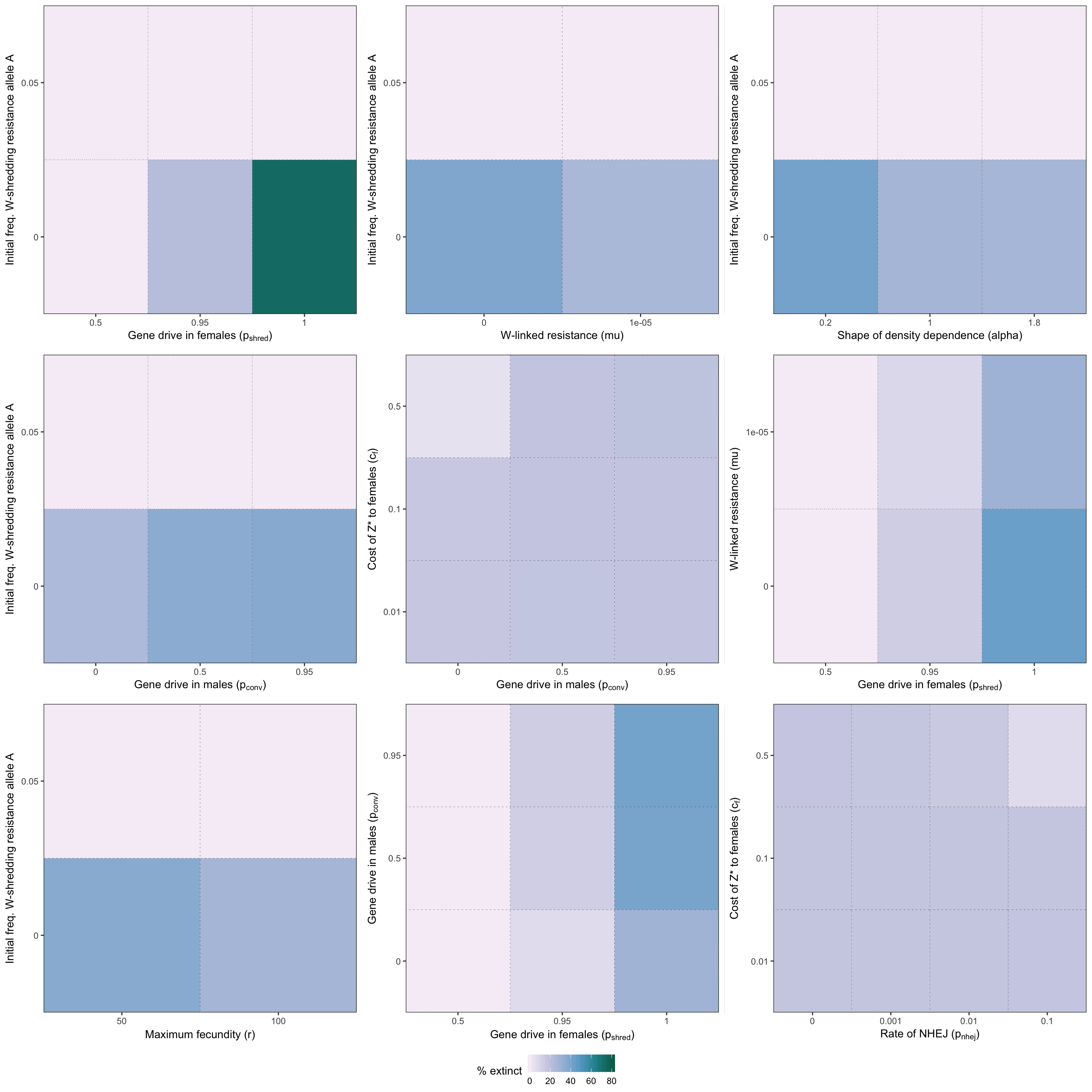Version Author Date
80ecff7 lukeholman 2019-05-07
9c1e80f lukeholman 2019-05-06

Figure S2: Heatmap illustrating the twelve strongest two-way interactions for simulations of a W-shredder, as determined by the effect sizes from the GLM plotted in Figure 4. The colour shows the % simulations runs that ended in extinction for each pair of parameter values.

grid.draw(two_way_plot_sterilising)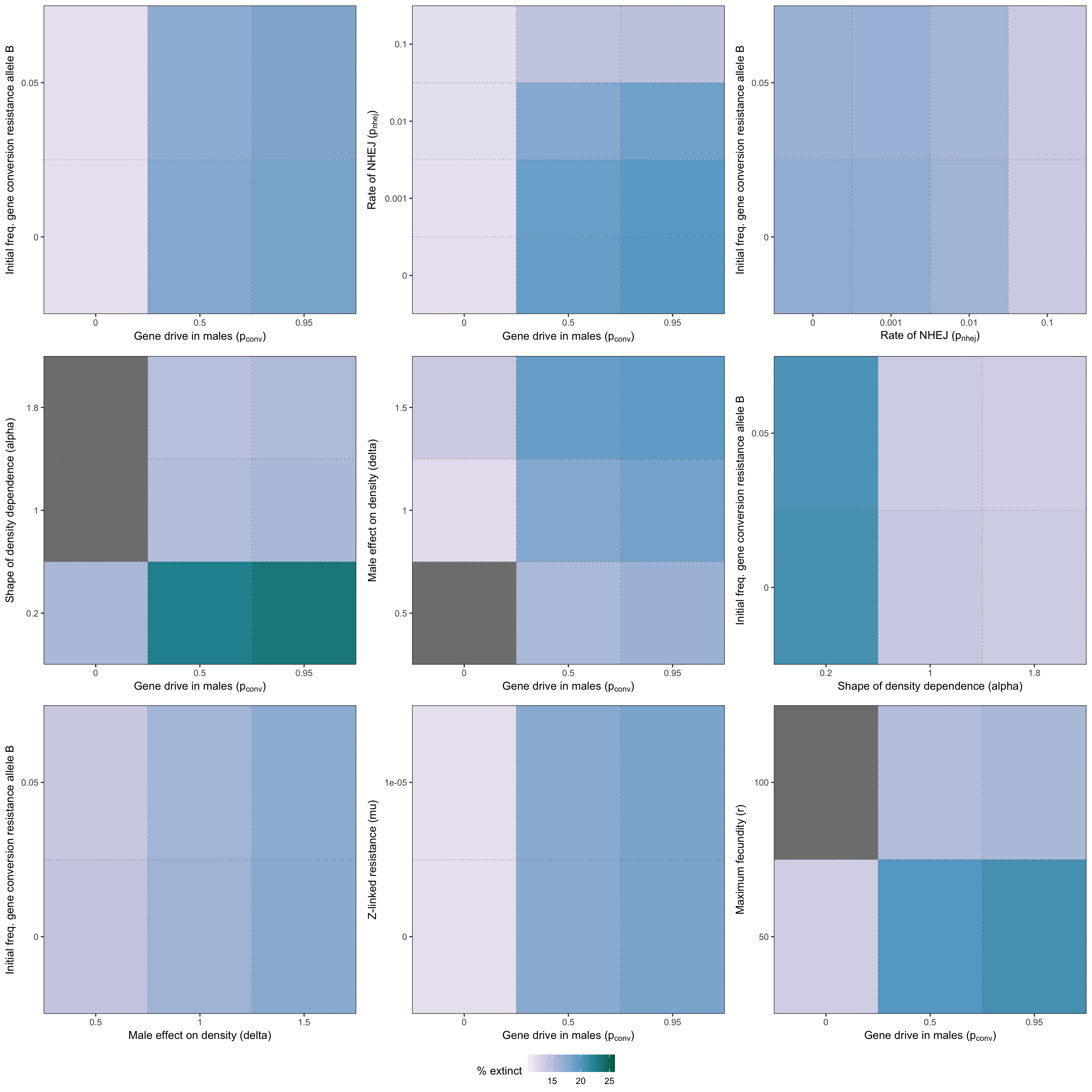Figure S6: Heatmap illustrating the twelve strongest two-way interactions for simulations of a female-sterilising gene drive, as determined by the effect sizes from the GLM plotted in Figure S5. The colour shows the % simulations runs that ended in extinction for each pair of parameter values.

sessionInfo()
R version 3.5.1 (2018-07-02)
Platform: x86_64-apple-darwin15.6.0 (64-bit)
Running under: macOS High Sierra 10.13.6

Matrix products: default
BLAS: /Library/Frameworks/R.framework/Versions/3.5/Resources/lib/libRblas.0.dylib
LAPACK: /Library/Frameworks/R.framework/Versions/3.5/Resources/lib/libRlapack.dylib

locale:
 en_AU.UTF-8/en_AU.UTF-8/en_AU.UTF-8/C/en_AU.UTF-8/en_AU.UTF-8

attached base packages:
 grid      parallel  stats     graphics  grDevices utils     datasets
 methods   base

other attached packages:
 future.apply_1.0.1 future_1.11.1.1    kableExtra_0.9.0
 biglm_0.9-1        DBI_1.0.0          data.table_1.12.2
 ggthemes_4.0.1     gridExtra_2.3      pander_0.6.2
 latex2exp_0.4.0    knitr_1.22         Cairo_1.5-9
 rslurm_0.4.0       Rcpp_1.0.1         reshape2_1.4.3
 stringr_1.4.0      tidyr_0.8.2        purrr_0.3.2
 dplyr_0.8.0.1

loaded via a namespace (and not attached):
 tidyselect_0.2.5   xfun_0.6           listenv_0.7.0
 colorspace_1.3-2   htmltools_0.3.6    viridisLite_0.3.0
 yaml_2.2.0         utf8_1.1.4         rlang_0.3.4
 pillar_1.3.1.9000  glue_1.3.1.9000    withr_2.1.2
 RColorBrewer_1.1-2 plyr_1.8.4         munsell_0.5.0
 gtable_0.2.0       workflowr_1.3.0    rvest_0.3.2
 codetools_0.2-15   evaluate_0.13      labeling_0.3
 fansi_0.4.0        scales_1.0.0       backports_1.1.2
 fs_1.3.1           hms_0.4.2          digest_0.6.18
 stringi_1.4.3      rprojroot_1.3-2    cli_1.1.0
 tools_3.5.1        magrittr_1.5       lazyeval_0.2.2
 crayon_1.3.4       whisker_0.3-2      pkgconfig_2.0.2
 xml2_1.2.0         assertthat_0.2.1   rmarkdown_1.10
 httr_1.4.0         rstudioapi_0.10    R6_2.4.0
 globals_0.12.4     git2r_0.23.0       compiler_3.5.1